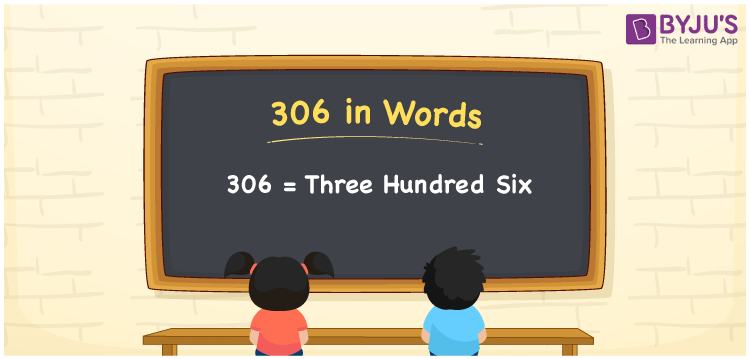# 306 in Words

306 in words can be written as Three Hundred Six. If you buy an ice cream tub for Rs. 306, then you can say that “I bought an ice cream tub for Three Hundred Six Rupees”. For a better understanding of the numbers in words concept, students can refer to the study materials provided at BYJU’S. Hence, 306 can be read as “Three Hundred Six” in words.

 306 in words Three Hundred Six Three Hundred Six in Numbers 306

## 306 in English Words## How to Write 306 in Words?

As mentioned above, students will be able to understand the method of creating the place value chart here.

 Hundreds Tens Ones 3 0 6

The expanded form of 306 is provided below:

3 x Hundred + 0 × Ten + 6 × One

= 3 x 100 + 0 x 10 + 6 x 1

= 300 + 6

= 306

= Three Hundred Six

Therefore, 306 in words is written as Three Hundred Six.

306 is a natural number that precedes 307 and succeeds 305.

306 in words – Three Hundred Six

Is 306 an odd number? – No

Is 306 an even number? – Yes

Is 306 a perfect square number? – No

Is 306 a perfect cube number? – No

Is 306 a prime number? – No

Is 306 a composite number? – Yes

## Frequently Asked Questions on 306 in Words

Q1

### How do you write the number 306 in words?

306 in words is Three Hundred Six.
Q2

### Find the value of 310 minus 4.

The value of 310 minus 4 is 306. Therefore, 306 in words is Three Hundred Six.
Q3

### Is 306 a perfect square?

No, 306 is not a perfect square as it cannot be expressed as the product of two integers which are equal.